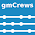### The Guided Cantilever Method - Part 2

The Guided Cantilever Method for Quickly Checking Piping Flexibility -- Part 2

This is a continuation of my last post. Here in Part 2, let's go through a specific example of how to do a quick manual check that a piping system has adequate flexibility to absorb required thermal expansion displacements.
The system consists of carbon steel pipe with a shorter leg of 20', an elbow, and a longer leg of 25'. The pipe's outside diameter is 6.625" (NPS 6"). And the fluid's operating temperature is 500F. As the pipe warms, the thermal expansion of both legs will generate enormous forces unless the system contains adequate flexibility to relieve the imposed displacements. Assuming the end anchors are rigid and as strong as the pipe (definitely not the case if attached to rotating equipment), controlling will be the expansion of the longer leg being absorbed by bending in the shorter leg. If the bending stresses in the shorter leg are below code allowables, then the system will be adequately flexible.

The first step is to conservatively estimate the free expansion of the longer leg.

The thermal expansion of pipe (y) in inches per 100 feet (in/100ft), between 70 degrees F and some temperature (T) can be approximated by:
``````y_per_100ft = (T - 100.) / 100.  // for carbon steel
y_per_100ft = 1.33 * (T - 100.) / 100.  // for stainless steel
``````
Or in words: For carbon steel, take the design temperature and subtract 100 then divide by 100. That's the expansion in inches per hundred feet. For stainless steel, add a third more.

The formulas will break down for temperatures around 200F or less. But for the range of temperatures commonly encountered, the equations overestimate less than 10 percent. And the formulas are easy to remember and work out in our head. (This is important if we want to be able to do quick manual checks.)

The next step is to conservatively calculate the length of the shorter leg needed to adequately absorb the expansion of the longer leg. For this we use a convenient version of the guided cantilever formula:
``````from math import sqrt

L = sqrt(E * y * D / S / 48)

// Where:
//    L = Length of cantilever beam (ft)
//    E = Youngs modulus (psi)
//    y = displacement of the the guided end (in)
//    D = beam (pipe) outside diameter (in)
//    S = max. nominal bending stress (psi)
``````
Modeling the shorter pipe leg as a cantilever whose guided end is being displaced by the free thermal expansion of the longer leg is a very conservative model for maximum bending stresses in Figure 1. For use with a calculator (remember those?) or an iPython session this formula can be made even simpler. Let:
• E=29E6. This is a typical maximum value for carbon steel. Stainless is less. So a conservative value to use for all materials.
• D=NPS. This is not a conservative assumption, but who can remember the outside diameters for all different nominal pipe sizes? I never could. This introduces an error of less than about 5%, which is more than made up for in the following assumption.
• S=10E3. Why such a conservatively low a number? (In the Peng link I gave in Part 1, the author used 20,000 psi for his guided cantilever method.) My justification for using 10,000 psi is:
• If the system lacks adequate flexibility, fatigue cracking will occur in the elbow, not the pipe. That's because the elbow has a "stress intensification factor" (SIF) associated with it. SIFs are multiplied by the nominal stress in the adjacent pipe to estimate the actual stress in the elbow.
• It is true that SIFs are mitigated by an elbow's "flexibility factor" (FF). (An elbow goes out-of-round under stress and so will strain more than simple beam theory would predict.) However, the stress reduction of FFs do not completely offset the stress intensification caused by SIFs. So, conservatively, elbow FFs are ignored.
• All pipe fittings, not just elbows, have SIFs associated with them. Piping fatigue almost always occurs in the system's fittings.
• SIFs are usually between 2.0 and 3.0.
• Finally, since thermal cycling causes fatigue failure, allowable fatigue stresses are about 1.5 times yield stress, which is typically between 20,000 and 30,000 psi. So using 10,000 psi, assuming a 2.0 to 3.0 SIF, and ignoring FFs will keep me 50% below a 1.5 times yield stress number.
• Therefore, using S=10000 for both carbon and stainless pretty much guarantees a conservative result. I can't recall ever encountering a pathological case where using this assumption gave an unconservative result.
The resulting formula is:
``````L = 7.77 * sqrt(y * NPS)  // 7.77 is easy to remember!

// Where:
//    y = thermal expansion to be absorbed (in)
//    NPS = nominal pipe size (in)
``````
Using this formula for the system in Figure 1 we have:
``````// Given:
// Expansion of longer leg which is 25 ft long:
y = ((500. - 100.) / 100.) * (25. / 100.)
// y = 1. (in)
// And nominal pipe size:
NPS = 6

// Required length of shorter leg:
L = 7.77 * sqrt(1. * 6.)
// L = 19. (ft)
``````
Since the shorter leg is 20 ft in length, the piping system is adequate.

#### 1 comment:

1.This is one of four posts on the guided cantilever method:

Part 1
Part 2
Part 3
Part 4